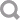eBay Product APIVersion 1.4.0# NumericValue

This class holds a numeric value

Type that uses NumericValue:

Calls that use NumericValue:

## Fields

unitOfMeasurement ( token ) [0..1]
Unit of measure for a numeric value.
Allowed values:
bps
Bits per second. A unit of data transmission.
kbps
Kilobits per second. A unit of data transmission.
bit
Binary digit. A unit of information storage.
byte
An ordered collection of bits. A unit of information storage equal to 8 bits in most contexts.
kb
Kilobit. A unit of information storage equal to 1024 bits.
MB
Megabyte. A unit of information storage equal to one million bytes.
mW
Megawatt. A unit of power equal to one million watts.
W
Watt. The base SI unit of power.
Hz
Hertz. A unit of frequency equal to one cycle per second.
kHz
Kilohertz. A unit of frequency equal to 1,000 cycles per second.
m
Meter. The base unit of distance.
mm
Megameter. A unit of distance equal to one million meters.
rph
Revolutions per hour. A unit of frequency.
hr
Hour. A unit of time equal to 3,600 seconds.
min
Minute. A unit of time equal to 60 seconds.
ms
Microsecond. A unit of time equal to one millionth of a second.
ns
Nanosecond. A unit of time equal to 10-9 seconds.
sec
Seconds. The base unit of time.
g
Grams. A unit of mass equal to one one-thousandth of the SI base unit, the kilogram.
lbs
Pounds. The base Imperial unit of mass.
The allowed units of measure do not apply to all numeric values. For example, when specifying the number of doors, unitOfMeasurement is not needed.
See the Field Index to learn which calls use unitOfMeasurement.

value ( string ) 
A numeric value.
See the Field Index to learn which calls use value.